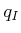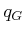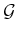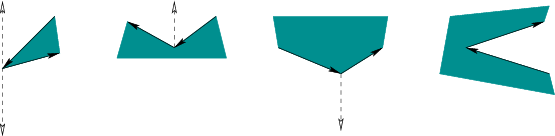## 6.2.2 Vertical Cell Decomposition

Cell decompositions will be defined formally in Section 6.3, but here we use the notion informally. Combinatorial methods must construct a finite data structure that exactly encodes the planning problem. Cell decomposition algorithms achieve this partitioning ofinto a finite set of regions called cells. The term-cell refers to a-dimensional cell. The cell decomposition should satisfy three properties:

1. Computing a path from one point to another inside of a cell must be trivially easy. For example, if every cell is convex, then any pair of points in a cell can be connected by a line segment.
2. Adjacency information for the cells can be easily extracted to build the roadmap.
3. For a givenand, it should be efficient to determine which cells contain them.
If a cell decomposition satisfies these properties, then the motion planning problem is reduced to a graph search problem. Once again the algorithms of Section 2.2 may be applied; however, in the current setting, the entire graph,, is usually known in advance.6.3 This was not assumed for discrete planning problems.Subsections
Steven M LaValle 2012-04-20